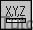# How to use Inquiry Tools For Points

## Here is how to use Inquiry Tools For Points in AutoCAD

You can use the Id command to find coordinates and this tool is usually used with an osnap.

• for example, you might want to find the coordinates for the center of an existing CIRCLE.
• you would invoke Id and then use a Center osnap to select the desired CIRCLE.Command: ‘_id Specify point: CEN
of (select CIRCLE)
X = 3.0000 Y = 3.0000 Z = 0.0000

You can use Dist to find the distance between two points and this tool is also normally used with an object snap.

• for example, you might want to find the distance between the centers of two existing CIRCLEs.
• you would invoke Id and then use a Center osnap to select the desired CIRCLEs.

Command: ‘_dist
Specify first point: CEN
of (select first CIRCLE)
Specify second point: CEN ↵
of (select second CIRCLE)
Distance = 6.0000, Angle in XY Plane = 0, Angle from XY Plane = 0
Delta X = 6.0000, Delta Y = 0.0000, Delta Z = 0.0000
Command: